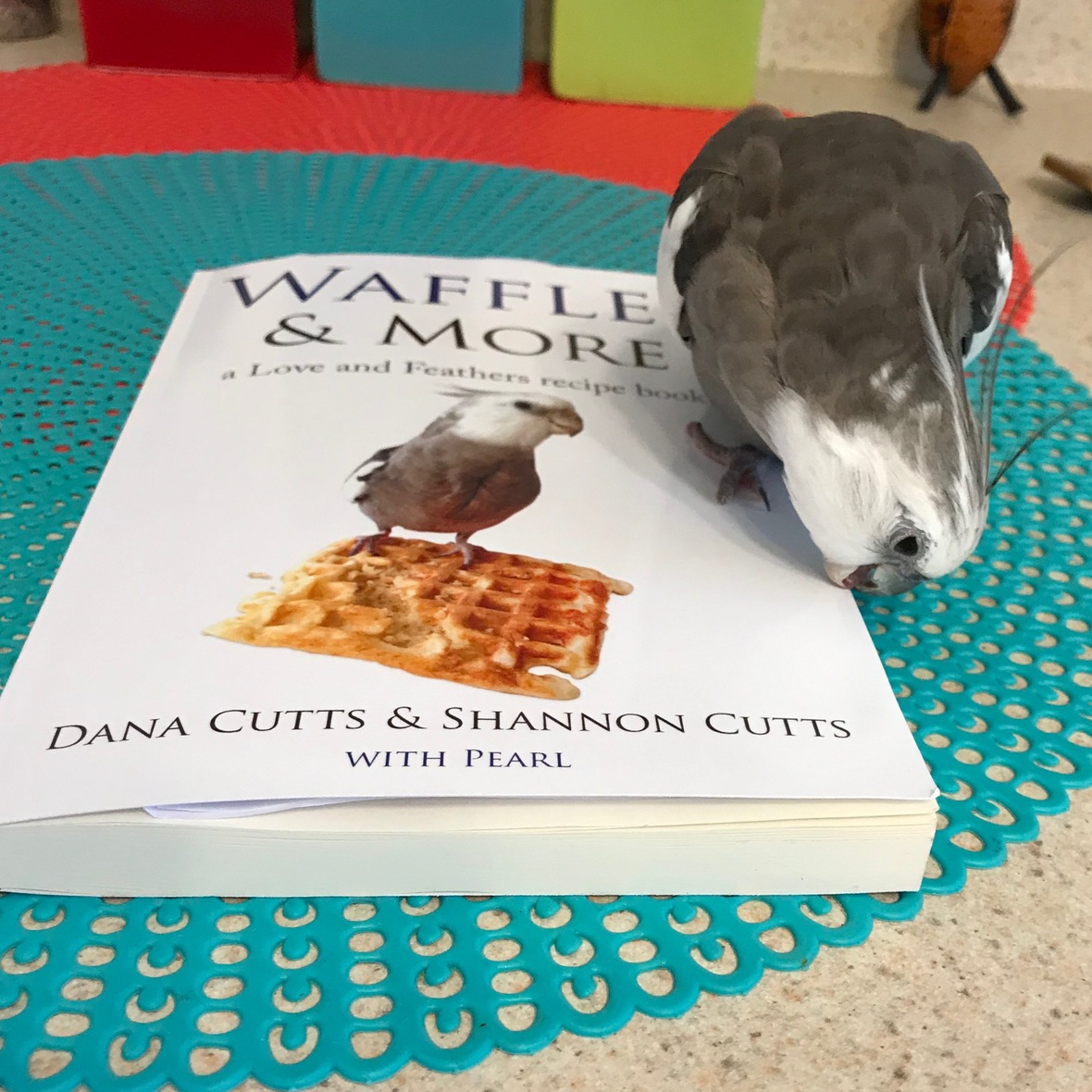Like   Tweet   Pin   +1   in

# Love & Feathers & Shells & Me

## November 2019

 table div table+table+table div table{width:100%;padding:0}table div table+table+table div table img{width:96.23%;padding:0;float:none}table div table+table+table div table td{width:100%;padding:0 1.88% 18px}/* styles *//* styles */ Receiving this email as a forward? Sign up to keep following our little flock's adventures!
 table div table+table+table+table+table+table div table{width:100%;padding:0}table div table+table+table+table+table+table div table img{width:96.23%;padding:0;float:none}table div table+table+table+table+table+table div table td{width:100%;padding:0 1.88% 18px}/* styles */table.module-6{width:75.66%;padding:0}table div table+table+table+table+table+table+table div table{width:75.66%;float:none;margin-left:auto;margin-right:auto;padding:0}table div table+table+table+table+table+table+table div table a{border:0 none;text-decoration:none}table div table+table+table+table+table+table+table div table img{width:100%!important;border:0 none;text-decoration:none}table div table+table+table+table+table+table+table div table td{width:100%;padding:0}/* styles */

## Flock news from the large featherless being....

 table div table+table+table+table+table+table+table+table+table div table,table.module-8{width:24.91%;float:right;padding:0}table div table+table+table+table+table+table+table+table+table div table a{border:0 none;text-decoration:none}table div table+table+table+table+table+table+table+table+table div table img{width:100%!important;border:0 none;text-decoration:none}table div table+table+table+table+table+table+table+table+table div table td{width:100%;padding:0 0 20px 20px}/* styles */ So here we are, poised to watch November magically turn into December. Wait for it. WIth the month o' gratitude even now inching away and the month o' gifts tiptoeing up behind it, there is a lot to love and a lot to miss. Speaking of missing, one member of our little flock is completely down for the count at this point, snoring the season away until the big round yellow heater in the sky gets its batteries changed and comes back on duty already. Mommy misses Bruce. Snif. If you've been following the flockwide Instagram, you know that a certain young lady recently got her own tortoise door. Malti's mommy installed it on her (formerly escape-proof) indoor winter habitat, baited it with edibles.... ....and unleashed the Kraken. "Coming out" has gone smooth and easy from the start. "Going back in"....well, that maneuver is still set to 'manual' for the time being. Meanwhile, our petite alpha flock member and his personal pint-sized birdie warmer have taken up right where they left off last winter. With lighter flock supervision duties and extra napping hours, not to mention all the holiday victuals that need sampling, Pearl is looking (and feeling) particularly full-feathered and fabulous. Of course. Thank goodness. As for the official large featherless/shell-less assistant, aka me, tis the season for turning a whole year older....almost. That big event will happen next month. We are all really looking forward to it, mostly because it is on this once-per-year occasion when the Small Chef makes one of her many memorable signature dishes..... Birthday cake. YUM!

==========

## Pearl, Malti, Bruce & Shannon

 table div table+table+table+table+table+table+table+table+table+table+table div table{width:100%;padding:0}table div table+table+table+table+table+table+table+table+table+table+table div table img{width:96.23%;padding:0;float:none}table div table+table+table+table+table+table+table+table+table+table+table div table td{width:100%;padding:0 1.88% 18px}/* styles */table.module-11{width:98.3%;padding:0}table div table+table+table+table+table+table+table+table+table+table+table+table div table{width:98.3%;float:none;margin-left:auto;margin-right:auto;padding:0}table div table+table+table+table+table+table+table+table+table+table+table+table div table a{border:0 none;text-decoration:none}table div table+table+table+table+table+table+table+table+table+table+table+table div table img{width:100%!important;border:0 none;text-decoration:none}table div table+table+table+table+table+table+table+table+table+table+table+table div table td{width:100%;padding:0}/* styles */
 table div table+table+table+table+table+table+table+table+table+table+table+table+table div table td,table.module-12{width:100%;padding:0}table div table+table+table+table+table+table+table+table+table+table+table+table+table div table{width:100%;float:none;margin-left:auto;margin-right:auto;padding:0}table div table+table+table+table+table+table+table+table+table+table+table+table+table div table a{border:0 none;text-decoration:none}table div table+table+table+table+table+table+table+table+table+table+table+table+table div table img{width:100%!important;border:0 none;text-decoration:none}/* styles */
 table div table+table+table+table+table+table+table+table+table+table+table+table+table+table+table div table{width:100%;padding:0}table div table+table+table+table+table+table+table+table+table+table+table+table+table+table+table div table img{width:96.23%;padding:0;float:none}table div table+table+table+table+table+table+table+table+table+table+table+table+table+table+table div table td{width:100%;padding:0 1.88% 18px}/* styles */# WIN A FREE COPY OF "LOVE & FEATHERS!"

==========

## Haven't read "Love & Feathers" yet?

The winner of "Love & Feathers" (print book version) will be chosen using a random number generator and notified via email on or before November 1.

## Good luck! :-)

 table div table+table+table+table+table+table+table+table+table+table+table+table+table+table+table+table+table+table div table{width:100%;padding:0}table div table+table+table+table+table+table+table+table+table+table+table+table+table+table+table+table+table+table div table img{width:96.23%;padding:0;float:none}table div table+table+table+table+table+table+table+table+table+table+table+table+table+table+table+table+table+table div table td{width:100%;padding:0 1.88% 18px}/* styles */table div table+table+table+table+table+table+table+table+table+table+table+table+table+table+table+table+table+table+table div table td,table.module-18{width:100%;padding:0}table div table+table+table+table+table+table+table+table+table+table+table+table+table+table+table+table+table+table+table div table{width:100%;float:none;margin-left:auto;margin-right:auto;padding:0}table div table+table+table+table+table+table+table+table+table+table+table+table+table+table+table+table+table+table+table div table a{border:0 none;text-decoration:none}table div table+table+table+table+table+table+table+table+table+table+table+table+table+table+table+table+table+table+table div table img{width:100%!important;border:0 none;text-decoration:none}/* styles */

# FREE Beak-o-graphs with every order (+ 1 extra-crispy cover)table div table+table+table+table+table+table+table+table+table+table+table+table+table+table+table+table+table+table+table+table+table+table+table div table{width:100%;padding:0}table div table+table+table+table+table+table+table+table+table+table+table+table+table+table+table+table+table+table+table+table+table+table+table div table img{width:96.23%;padding:0;float:none}table div table+table+table+table+table+table+table+table+table+table+table+table+table+table+table+table+table+table+table+table+table+table+table div table td{width:100%;padding:0 1.88% 18px}/* styles */# The pages are pretty delectable too....

 table.module-24{width:75.47%;padding:0}table div table+table+table+table+table+table+table+table+table+table+table+table+table+table+table+table+table+table+table+table+table+table+table+table+table div table{width:75.47%;float:none;margin-left:auto;margin-right:auto;padding:0}table div table+table+table+table+table+table+table+table+table+table+table+table+table+table+table+table+table+table+table+table+table+table+table+table+table div table a{border:0 none;text-decoration:none}table div table+table+table+table+table+table+table+table+table+table+table+table+table+table+table+table+table+table+table+table+table+table+table+table+table div table img{width:100%!important;border:0 none;text-decoration:none}table div table+table+table+table+table+table+table+table+table+table+table+table+table+table+table+table+table+table+table+table+table+table+table+table+table div table td{width:100%;padding:0}/* styles */
 table div table+table+table+table+table+table+table+table+table+table+table+table+table+table+table+table+table+table+table+table+table+table+table+table+table+table+table div table{width:100%;padding:0}table div table+table+table+table+table+table+table+table+table+table+table+table+table+table+table+table+table+table+table+table+table+table+table+table+table+table+table div table img{width:96.23%;padding:0;float:none}table div table+table+table+table+table+table+table+table+table+table+table+table+table+table+table+table+table+table+table+table+table+table+table+table+table+table+table div table td{width:100%;padding:0 1.88% 18px}/* styles */# Hibernation hay - it's the coziest!!

 table.module-28{width:75.47%;padding:0}table div table+table+table+table+table+table+table+table+table+table+table+table+table+table+table+table+table+table+table+table+table+table+table+table+table+table+table+table+table div table{width:75.47%;float:none;margin-left:auto;margin-right:auto;padding:0}table div table+table+table+table+table+table+table+table+table+table+table+table+table+table+table+table+table+table+table+table+table+table+table+table+table+table+table+table+table div table a{border:0 none;text-decoration:none}table div table+table+table+table+table+table+table+table+table+table+table+table+table+table+table+table+table+table+table+table+table+table+table+table+table+table+table+table+table div table img{width:100%!important;border:0 none;text-decoration:none}table div table+table+table+table+table+table+table+table+table+table+table+table+table+table+table+table+table+table+table+table+table+table+table+table+table+table+table+table+table div table td{width:100%;padding:0}/* styles */
 table div table+table+table+table+table+table+table+table+table+table+table+table+table+table+table+table+table+table+table+table+table+table+table+table+table+table+table+table+table+table+table div table{width:100%;padding:0}table div table+table+table+table+table+table+table+table+table+table+table+table+table+table+table+table+table+table+table+table+table+table+table+table+table+table+table+table+table+table+table div table img{width:96.23%;padding:0;float:none}table div table+table+table+table+table+table+table+table+table+table+table+table+table+table+table+table+table+table+table+table+table+table+table+table+table+table+table+table+table+table+table div table td{width:100%;padding:0 1.88% 18px}/* styles */## Meet Pearl, Malti, Bruce & Me

 table div table+table+table+table+table+table+table+table+table+table+table+table+table+table+table+table+table+table+table+table+table+table+table+table+table+table+table+table+table+table+table+table+table div table,table.module-32{width:34.34%;float:left;padding:0}table div table+table+table+table+table+table+table+table+table+table+table+table+table+table+table+table+table+table+table+table+table+table+table+table+table+table+table+table+table+table+table+table+table div table a{border:0 none;text-decoration:none}table div table+table+table+table+table+table+table+table+table+table+table+table+table+table+table+table+table+table+table+table+table+table+table+table+table+table+table+table+table+table+table+table+table div table img{width:100%!important;border:0 none;text-decoration:none}table div table+table+table+table+table+table+table+table+table+table+table+table+table+table+table+table+table+table+table+table+table+table+table+table+table+table+table+table+table+table+table+table+table div table td{width:100%;padding:0 20px 20px 0}/* styles */ Hi! It is so good to meet you here each month! Pearl, Malti, Bruce and their mommy love having you in our extended flock! We are super excited about our newest book, "Waffles & More: A Love & Feathers Recipe Book, which features 70 of Pearl's favorite "people food" recipes, courtesy of his personal Small Chef (aka his doting large featherless Grandma). Have you treated yourself yet to a copy of "Love & Feathers: what a palm-sized parrot has taught me about life, love, and healthy self-esteem?" If not, it's time! Get ready to smile, laugh, and fall in love with your own pets all over again. ==> To connect with me: www.shannoncutts.com ==> To connect with Pearl, Malti & Bruce: www.loveandfeathersandshells.com
 table div table+table+table+table+table+table+table+table+table+table+table+table+table+table+table+table+table+table+table+table+table+table+table+table+table+table+table+table+table+table+table+table+table+table div table{width:100%;padding:0}table div table+table+table+table+table+table+table+table+table+table+table+table+table+table+table+table+table+table+table+table+table+table+table+table+table+table+table+table+table+table+table+table+table+table div table img{width:96.23%;padding:0;float:none}table div table+table+table+table+table+table+table+table+table+table+table+table+table+table+table+table+table+table+table+table+table+table+table+table+table+table+table+table+table+table+table+table+table+table div table td{width:100%;padding:0 1.88% 18px}/* styles */table div table+table+table+table+table+table+table+table+table+table+table+table+table+table+table+table+table+table+table+table+table+table+table+table+table+table+table+table+table+table+table+table+table+table+table div table td,table.module-34{width:100%;padding:0}table div table+table+table+table+table+table+table+table+table+table+table+table+table+table+table+table+table+table+table+table+table+table+table+table+table+table+table+table+table+table+table+table+table+table+table div table{width:100%;float:none;margin-left:auto;margin-right:auto;padding:0}table div table+table+table+table+table+table+table+table+table+table+table+table+table+table+table+table+table+table+table+table+table+table+table+table+table+table+table+table+table+table+table+table+table+table+table div table a{border:0 none;text-decoration:none}table div table+table+table+table+table+table+table+table+table+table+table+table+table+table+table+table+table+table+table+table+table+table+table+table+table+table+table+table+table+table+table+table+table+table+table div table img{width:100%!important;border:0 none;text-decoration:none}/* styles */
 table div table+table+table+table+table+table+table+table+table+table+table+table+table+table+table+table+table+table+table+table+table+table+table+table+table+table+table+table+table+table+table+table+table+table+table+table div table{width:100%;padding:0}table div table+table+table+table+table+table+table+table+table+table+table+table+table+table+table+table+table+table+table+table+table+table+table+table+table+table+table+table+table+table+table+table+table+table+table+table div table img{width:96.23%;padding:0;float:none}table div table+table+table+table+table+table+table+table+table+table+table+table+table+table+table+table+table+table+table+table+table+table+table+table+table+table+table+table+table+table+table+table+table+table+table+table div table td{width:100%;padding:0 1.88% 18px}/* styles */